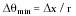Nanomedicine, Volume I: Basic Capabilities

Robert A. Freitas Jr., Nanomedicine, Volume I: Basic Capabilities, Landes Bioscience, Georgetown, TX, 1999

4.3.4.2 Nanopendulum Orientation Sensing

A nanopendulum may also be employed for orientational sensing. As one example of a large class of related devices, consider a simple rigid spherical nanopendulum of radius r and mass m, with a large hemispherical bob free to swing through the entire solid angle about a central pivot point (Fig. 4.5), with a gimballed housing to allow active avoidance of the pivot support beam. The nanopendulum bob moves along a spherical surface located far enough from a concentric spherical sensor grid of radius R = r + zsep to minimize van der Waals interactions (Sections 3.5.1 and 9.2), with bob and sensor surfaces electrically neutral or uniformly mutually repulsive. Much like the pin cushion receptor model (Section 3.5.7.4), the sensor grid consists of a spherical array of diamondoid sensor rods Dx ~ 1 nm wide that are rapidly and simultaneously extended into the evacuated sensor cavity to determine bob position during a measurement cycle of duration tmeas. Radial sensor rod velocity should exceed typical tangential bob velocity by at least 1-2 orders of magnitude. For the example given below, a sensor stud on a 500-nm bob that is turning at vturn ~ vthermal = 3.6 mm/sec passes a ~1 nm sensor rod in ~280 nanosec; a sensor rod displacing ~1 nm in the radial direction in ~1 nanosec travels at vrod ~ 1 m/sec. This nanopendulum may serve both as an orientation sensor and as a rotation rate sensor, using principles similar to vestibular (semicircular canal) mechanics.449

If a simple nanopendulum spherical sensor housing with bob initially at rest in a uniform vertical gravity field g is rotated through the smallest detectable angle qmin (radians) around the center pivot, then ignoring friction, the bob's position over the sensor grid moves a distance Dx, hence{Eqn. 4.22}

For Dx = 1 nm and r = 500 nm, Dqmin ~ 2 milliradians (~0.1 deg). For a practical sliding interface (e.g., between bob and sensor surface) of contact area S and turning velocity vturn, Drexler10 estimates frictional dissipation, primarily driven by shear-reflection drag and band-stiffness scattering, as Pdrag ~ k1 vturn2 S, where k1 ~ 400 kg/m2-sec, hence the energy dissipated between the bob and the nearest sensor rod (the one interacting with the bob) is Edrag ~ k1 vturn S Dx. Bob gravitational energy Ebob = m g r sin Dqmin ~ m g r Dqmin for small displacements. The bob swings freely at the minimum displacement if Ebob/Edrag >1. If m = 10-15 kg, r = 500 nm, and S = 1 nm2, then vturn < 2.5 m/sec or w < 49 megaradians/sec for the bob to swing freely (vs. vthermal = 3.6 mm/sec (Eqn. 3.3) and wres ~ 9900 rad/sec for this pendulum).

The bob-up/bob-down energy differential is ~2 m g r = 9.8 zJ ~2.3 kT for m = 10-15 kg and r = 500 nm. To distinguish opposite orientations (Dq ~ p) requires Nmeas ~ (kT / 2 m g r)2 ~ 1 measurement for this sensor. To distinguish Dqmin ~ 2 milliradians requires averaging Nmeas ~ (kT / Ebob)2 ~ 190,000 independent measurements (of ~0.002 kT/measurement) or tmeas ~ 190 microsec using a sensor rod clock cycle of ~1 nanosec. Thus, after subtracting out the effects of translation displacements as determined by linear accelerometers (Section 4.3.3), a nanodevice can measure angular displacements in its orientation to within ± Dqmin in a measurement time tmeas ~10-4 sec. With sensor rods moving at ~1 m/sec, energy dissipation for each rod sliding in its housing is ~0.01 zJ/cycle (Section 10.2.1). If only ~100 test rods need be triggered to continuously track the moving stud, then a sensor rod clock cycle of ~1 nanosec implies a continuous nanopendulum power dissipation of ~1 pW; additional drag power loss between stud and contacting rod is only Pdrag ~0.0004 pW.

Many other configurations of this basic device are readily conceived and may be more volume-efficient, including nested spheres or ovoids, two-dimensional rocking disk sections for in-plane rotational measurements (three devices required for monitoring all angular degrees of freedom in a rigid rotator), and multiaxial circumferential tracks or tubes containing rolling balls or sliding plugs. J. Logajan suggests that a pair of linear three-dimensional translation accelerometers should be able to unambiguously extract the 3 translation and 3 rotation components of all possible acceleration vectors. Rotation about any axis will result in distinctly different mass displacements while accelerated translation along any axis will result in identical displacements.

Last updated on 17 February 2003